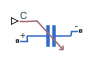# Variable Capacitor

Linear time-varying capacitor

•Libraries:
Simscape / Electrical / Passive

## Description

The Variable Capacitor block represents a linear time-varying capacitor. The block provides two options for the relationship between the current i through the capacitor and the voltage v across the device when the capacitance at port C is C. The Equation parameter determines which of the following equations the block uses:

• $i=C\frac{dv}{dt}$

Use the preceding equation when the capacitance is defined as the local gradient of the charge-voltage curve for a given voltage:

`$C\left(v\right)=\frac{dQ\left(v\right)}{dv}$`

• $i=\frac{dC}{dt}v+C\frac{dv}{dt}$

Use the preceding equation when the capacitance is defined as the ratio of the charge Q to the steady-state voltage:

`$C\left(v\right)=\frac{Q\left(v\right)}{v}$`

The block includes a resistor in series with the variable capacitor. You can use this resistor to represent the total ohmic connection resistance of the capacitor. You may need to use this resistor to prevent numerical issues for some circuit topologies, such as where a Variable Capacitor block is connected in parallel with another capacitor block that does not have a series resistance.

## Ports

### Input

expand all

Physical signal input port associated with the capacitance. C must be finite and greater than zero

### Conserving

expand all

Electrical conserving port associated with the capacitor positive voltage.

Electrical conserving port associated with the capacitor negative voltage.

## Parameters

expand all

Select one of the following options for block capacitance:

• `I = C*dV/dt` — This equation assumes the capacitance is defined as the local gradient of the charge-voltage curve for a given voltage. This option is the default.

• `I = C*dV/dt + dC/dt*V` — This equation assumes the capacitance is defined as the ratio of the charge to the steady-state voltage.

The lower limit on the value of the signal at port C. This limit prevents the signal from reaching a value that has no physical meaning. The default value is `1e-09` F.

The value of the resistance placed in series with the variable capacitor. The default value is `1e-06` Ohm.

The output voltage at the start of the simulation.

#### Dependencies

This parameter is visible only when you select ```I = C*dV/dt``` for the Equation parameter.

The charge at the start of the simulation.

#### Dependencies

This parameter is visible only when you select ```I = C*dV/dt + dC/dt*V``` for the Equation parameter.

## Version History

Introduced in R2008a